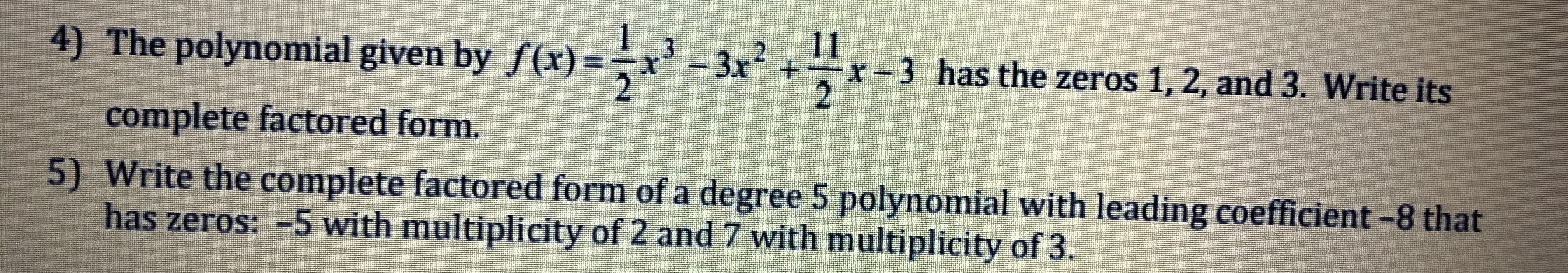# 4) The polynomial given by f(x) x2+r-3 has the zeros 1, 2, and 3. Write its complete factored form. 5) Write the complete factored form of a degree 5 polynomial with leading coefficient -8 that has zeros: -5 with multiplicity of 2 and 7 with multiplicity of 3.

Question

For #4help_outlineImage Transcriptionclose4) The polynomial given by f(x) x2+r-3 has the zeros 1, 2, and 3. Write its complete factored form. 5) Write the complete factored form of a degree 5 polynomial with leading coefficient -8 that has zeros: -5 with multiplicity of 2 and 7 with multiplicity of 3. fullscreen

### Want to see the step-by-step answer?